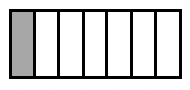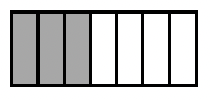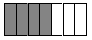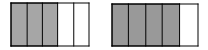### Home > AC > Chapter 11 > Lesson 11.3.1 > Problem11-104

11-104.The diagram below shows one way to represent the fraction $\frac { 1 } { 7 }$.

1. Draw a similar diagram to represent $\frac { 3 } { 7 }$.2. What is $\frac { 1 } { 7 } + \frac { 3 } { 7 }$? Use your diagram to justify your answer.$\frac { 1 } { 7 } + \frac { 3 } { 7 } = \frac { 4 } { 7 }$

3. Use a new diagram to add $\frac { 3 } { 5 } + \frac { 4 } { 5 }$.$\frac { 3 } { 5 } + \frac { 4 } { 5 } = \frac { 7 } { 5 }$